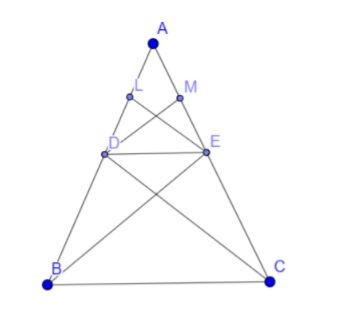QuestionAnswers

# State BPT theorem and prove it.

Hint: To prove this theorem first we will join BE and CD. Then draw a line EL perpendicular to AB and line DM perpendicular to AC. Now we will find the ratio of area of $\Delta$ADE to $\Delta$DBE and ratio of area of $\Delta$ADE to $\Delta$ECD. Comparing the ratios we will get the final answer.Now, $\Delta DBE$ and $\Delta ECD$ being on the same base DE and between the same parallels DE and BC, we have,$ar(\Delta DBE)=ar(\Delta ECD)$ then we say that the basic proportionality theorem is proved.

Basic proportionality theorem:
If a line is drawn parallel to one side of a triangle to intersect the other two sides in distinct points then the other two sides are divided in the same ratio.
Given:
A $\Delta ABC$in which $DE\parallel BC$and DE intersects AB and AC at D and E respectively.
To prove that:
$\dfrac{AD}{DB}=\dfrac{AE}{EC}$
Construction:
Join BE and CD.
Draw $EL\bot AB$and $DM\bot AC$
Proof:
We have the
$ar(\Delta ADE)=\dfrac{1}{2}\times AD\times EL$
$ar(\Delta DBE)=\dfrac{1}{2}\times DB\times EL$
Therefore the ratio of these two is $\dfrac{ar(\Delta ADE)}{ar(\Delta DBE)}=\dfrac{AD}{DB}$. . . . . . . . . . . . . . (1)
Similarly,
$ar(\Delta ADE)=ar(\Delta ADE)=\dfrac{1}{2}\times AE\times DM$
$ar(\Delta ECD)=\dfrac{1}{2}\times EC\times DM$
Therefore the ratio of these two is $\dfrac{ar(\Delta ADE)}{ar(\Delta ECD)}=\dfrac{AE}{EC}$. . . . . . . . . . . .. . . (2)

Now, $\Delta DBE$ and $\Delta ECD$ being on the same base DE and between the same parallels DE and BC, we have,
$ar(\Delta DBE)=ar(\Delta ECD)$. . . . . . . . . . . (3)
From equations 1, 2, 3 we can conclude that
$\dfrac{AD}{DB}=\dfrac{AE}{EC}$
Hence we can say that the basic proportionality theorem is proved.

Note: The formula for area of the triangle is given by $\dfrac{1}{2}\times b\times h$where b, h are base and height respectively. If two triangles are on the same base and between the same parallels then the area of those two triangles are equal.